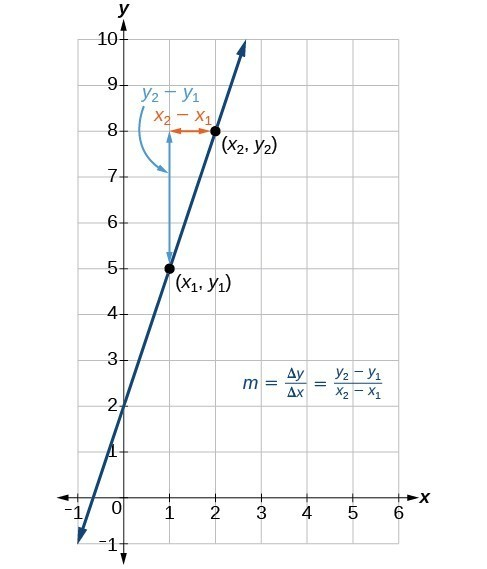## Calculate and interpret slope

In the examples we have seen so far, we have had the slope provided for us. However, we often need to calculate the slope given input and output values. Given two values for the input, ${x}_{1}$ and ${x}_{2}$, and two corresponding values for the output, ${y}_{1}$ and ${y}_{2}$ —which can be represented by a set of points, $\left({x}_{1}\text{, }{y}_{1}\right)$ and $\left({x}_{2}\text{, }{y}_{2}\right)$—we can calculate the slope $m$, as follows

$m=\frac{\text{change in output (rise)}}{\text{change in input (run)}}=\frac{\Delta y}{\Delta x}=\frac{{y}_{2}-{y}_{1}}{{x}_{2}-{x}_{1}}$

where $\Delta y$ is the vertical displacement and $\Delta x$ is the horizontal displacement. Note in function notation two corresponding values for the output ${y}_{1}$ and ${y}_{2}$ for the function $f$, ${y}_{1}=f\left({x}_{1}\right)$ and ${y}_{2}=f\left({x}_{2}\right)$, so we could equivalently write

$m=\frac{f\left({x}_{2}\right)-f\left({x}_{1}\right)}{{x}_{2}-{x}_{1}}$

The graph in Figure 5 indicates how the slope of the line between the points, $\left({x}_{1,}{y}_{1}\right)$
and $\left({x}_{2,}{y}_{2}\right)$, is calculated. Recall that the slope measures steepness. The greater the absolute value of the slope, the steeper the line is.Figure 5

The slope of a function is calculated by the change in $y$ divided by the change in $x$. It does not matter which coordinate is used as the $\left({x}_{2,\text{ }}{y}_{2}\right)$ and which is the $\left({x}_{1},\text{ }{y}_{1}\right)$, as long as each calculation is started with the elements from the same coordinate pair.

### Q & A

Are the units for slope always $\frac{\text{units for the output}}{\text{units for the input}}$ ?

Yes. Think of the units as the change of output value for each unit of change in input value. An example of slope could be miles per hour or dollars per day. Notice the units appear as a ratio of units for the output per units for the input.

### A General Note: Calculate Slope

The slope, or rate of change, of a function $m$ can be calculated according to the following:

$m=\frac{\text{change in output (rise)}}{\text{change in input (run)}}=\frac{\Delta y}{\Delta x}=\frac{{y}_{2}-{y}_{1}}{{x}_{2}-{x}_{1}}$

where ${x}_{1}$ and ${x}_{2}$ are input values, ${y}_{1}$ and ${y}_{2}$ are output values.

### How To: Given two points from a linear function, calculate and interpret the slope.

1. Determine the units for output and input values.
2. Calculate the change of output values and change of input values.
3. Interpret the slope as the change in output values per unit of the input value.

### Example 3: Finding the Slope of a Linear Function

If $f\left(x\right)$ is a linear function, and $\left(3,-2\right)$ and $\left(8,1\right)$ are points on the line, find the slope. Is this function increasing or decreasing?

### Solution

The coordinate pairs are $\left(3,-2\right)$ and $\left(8,1\right)$. To find the rate of change, we divide the change in output by the change in input.

$m=\frac{\text{change in output}}{\text{change in input}}=\frac{1-\left(-2\right)}{8 - 3}=\frac{3}{5}$

We could also write the slope as $m=0.6$. The function is increasing because $m>0$.

### Analysis of the Solution

As noted earlier, the order in which we write the points does not matter when we compute the slope of the line as long as the first output value, or y-coordinate, used corresponds with the first input value, or x-coordinate, used.

### Try It 1

If $f\left(x\right)$ is a linear function, and $\left(2,\text{ }3\right)$ and $\left(0,\text{ }4\right)$ are points on the line, find the slope. Is this function increasing or decreasing?

Solution

### Example 4: Finding the Population Change from a Linear Function

The population of a city increased from 23,400 to 27,800 between 2008 and 2012. Find the change of population per year if we assume the change was constant from 2008 to 2012.

### Solution

The rate of change relates the change in population to the change in time. The population increased by $27,800 - 23,400=4400$ people over the four-year time interval. To find the rate of change, divide the change in the number of people by the number of years.

$\frac{4,400\text{ people}}{4\text{ years}}=1,100\text{ }\frac{\text{people}}{\text{year}}$

So the population increased by 1,100 people per year.

### Analysis of the Solution

Because we are told that the population increased, we would expect the slope to be positive. This positive slope we calculated is therefore reasonable.

### Try It 2

The population of a small town increased from 1,442 to 1,868 between 2009 and 2012. Find the change of population per year if we assume the change was constant from 2009 to 2012.

Solution